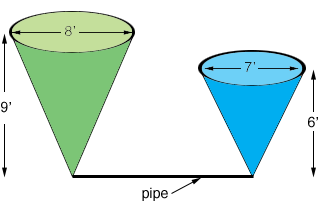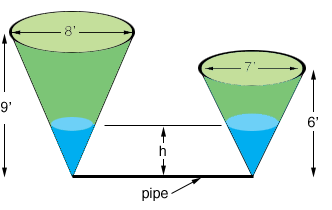SEARCH HOMEMath Central Quandaries & QueriesQuestion from rustom, a student: Two vertical conical tanks (both inverted) have their vertices connected by a short horizontal pipe. One tank, initially full of water, has an altitude of 6 ft. and a diameter of base 7 ft. The other tank, initially empty, has an altitude of 9 ft., and a diameter of base 8 ft. If the water is allowed to flow through the connecting pipe, find the level to which the water will ultimately rise in the empty tank (Neglect the water in the pipe.) I did not understand how the question works, whether the volume of the water from the smaller will all go to the bigger conical tank. Please explain what quantity is unknown in the question. Answer = 5.1510 ftInitially all the water is in the smaller tank.Once the water is allowed to flow through the pipe the atmospheric pressure forces the water to flow through the pipe until the system reaches an equilibrium state where the water in both tanks is the same height $h$.It is this height $h$ that you want.

Write back if you need more help,
PennyMath Central is supported by the University of Regina and The Pacific Institute for the Mathematical Sciences.